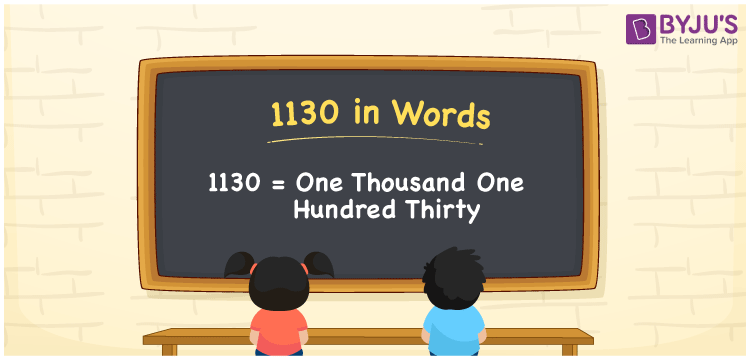# 1130 in Words

1130 in words is written as “One Thousand One Hundred Thirty”. In Maths, 1130 is a cardinal number that expresses a quantity or a value. If the cost of a bus ticket is Rs.1130, then it can be represented as Rupees One Thousand One Hundred Thirty only. Learn more about Numbers In Words at BYJU’S and how to spell 1130 in words, in this article.

 1130 in Words One Thousand One Hundred Thirty One Thousand One Hundred Thirty in Numerals 1130

## 1130 in English Words## How to Write 1130 in Words?

The number 1130 in words can be written using a place value method. Since 1130 is a four-digit number, hence, the place value of each digit is given by;

 Thousands Hundreds Tens Ones 1 1 3 0

From the above table,

1 → Thousands place

1 → Hundreds place

3 → Tens place

0 → Ones place

So, when we read the number from right to left, it is spelt as One Thousand One Hundred Thirty.

### Expanded Form of 1130

We can write the expanded form as:

1 x Thousand + 1 × Hundred + 3 × Ten + 0 × One

= 1 x 1000 + 1 × 100 + 3 × 10 + 0 × 1

= 1000 + 100 + 30 + 0

= 1130

= One Thousand One Hundred Thirty

1130 is a whole number that is succeeded by 1129 and preceded by 1131. Learn more about the number 1130 below:

• 1130 in Words – One Thousand One Hundred Thirty
• Is 1130 an odd number? – No
• Is 1130 an even number? – Yes
• Is 1130 a perfect square number? – No
• Is 1130 a perfect cube number? – No
• Is 1130 a prime number? – No
• Is 1130 a composite number? – Yes

## Frequently Asked Questions on 1130 in words

Q1

### What is 1130 in words?

1130 in words is given by One Thousand One Hundred Thirty.
Q2

### What is the rule to write 1130 in words?

1130 in words is written based on the place values of each digit. 1 is at thousands, 1 at hundreds, 3 at tens and 0 at ones. Thus, reading from right to left, it is written as One Thousand One Hundred Thirty.
Q3

### What is the value of 1130 plus 200 in words?

1130 + 200 = 1330, i.e., One Thousand Three Hundred Thirty in words
Test your Knowledge on 1130 in Words